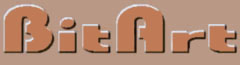Properties of Spirolaterals From the introductory example, the spirolateral is defined by three basic factors: the turning angle, the number of segments or turns, and the number of repetitions, which create a closed figure.  To investigate the closing property of a spirolateral, Figure 3 shows a 90 degree spirolateral from 1 to 10 turns.Figure 3. Spirolateral of 90 degrees, from 1 to 10 turns From the example, the spirolaterals of turns 4 and 8 do not close, all the others do.  To predict which parameters generate closed spirolaterals, we see from the following analysis that when the total turning angle is modulo 360 degrees, the spirolateral will be closed.  Total turning angle = angle of turns x number of turns x repetitions    a.   90  x  1  x  4  =   360      b.   90  x  2  x  2  =   360     c.   90  x  3  x  4  =  1080    d.   90  x  4  x  1  =  360    e.   90  x  5  x  4  =  1800     f.   90  x  6  x  2  =  1080     g.   90  x  7  x  4  =  2520     h.   90  x  8  x  1  =  360    i.    90  x  9  x  4  =  3240     j.   90  x 10  x  2 =  1800  The exception is that when only one repetition become 360 degrees, the spirolateral will not be closed, or if repeated further it will just meander outward without ever closing on itself.  Odds developed a notation to describe each of the spirolaterals.  The number of turns, n, referred to as the order of the spirolateral, is the base number.  The angle of turn is written as a subscript; thus, 590 would define a spirolateral with five turns each through 90 degrees.  Odds suspected that any angle that is an exact divisor of 180 degrees would generate a spirolateral, Figure 4 shows a series based on those angles.  Gardner also agreed with Odds without investigating any other possible angles.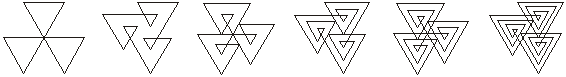260                        460                       560                           760                        860                         1060145                              245                      345                          545                         645                        1045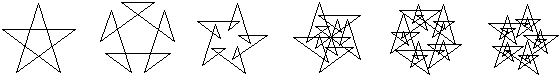136                          236                       336                           436                        736                        836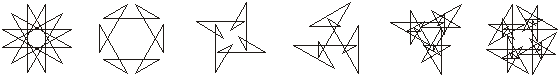130                          230                        330                           430                        830                        930 Figure 4. Spirolaterals based on an angle 180/n To test the 180/n observation, a computer program was written to generate all the possible spirolaterals of angles from 180/2 to 180/30 restricting them to a maximum of 10 turns and 10 repeats.  Of these 290 possible spirolaterals, 143 were closed by the 360 degree rule; of the 143, 10 were not actually closed. Another computer program was written to enumerate other spirolaterals.  The enumeration included all the angles from 1 to 180, incremented by 1 degree, and number of turns from 1 to 10 were attempted to generate a closed spirolateral, with a maximum of 10 repeats.  From 1800 possible spirolaterals, 561 were closed by the 360 degree rule; of the 561, 113 were not actually closed.  Of that gallery of 448 images, Figure 5 exhibits a representative selection.970                                            980                                         696                                                101008105                                         3108                                            8108                                           9110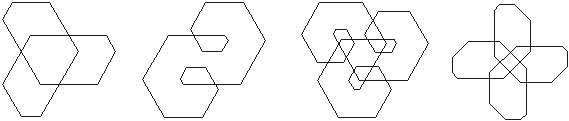4120                                        9120                                          10120                                     6135  Figure 5.  Spirolaterals based on angles not 180/n

BitArt, Robert J. Krawczyk
5120 W. Wilson Ave., Chicago, IL 60630
773-283-0313, bitart@ix.netcom.com